# How to use comparators for basic maths (add and substract)

• 2,178 views, 1 today
• 4
• 1
• 1
Basic notions:

The comparator is a redstone component which has two mode :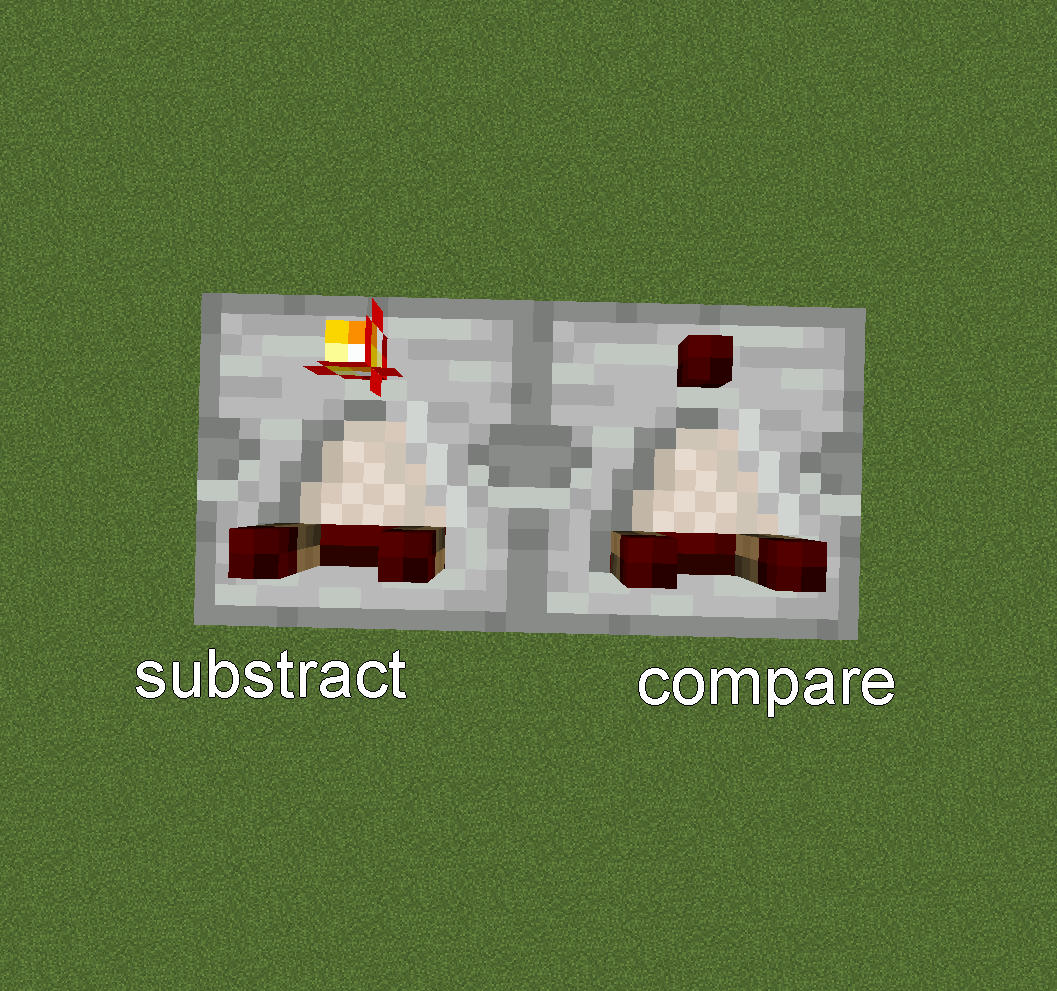- compare :
return input if control < input
else return 0
- substract :
return input - control

For example.
for input = 14 and control = 13 , a comparator returns 14 in compare mode and 1 in substract mode.

(note : you can use F3 to see the redstone wire power [at the right] )

--> by substracting !

Indeed , in maths A-(-B) = A+B

However in Minecraft if A = 2 and B = 10 , A-(-B) = 2-(0) = 2 because restone works on base16 (from 0 to 15).

How to fix that ?
--> a little trick

We know that 15 - 15 = 0 And A-(-B) = A+B.

So 15 - (15 - A - B) = 15 -15 + A + B = A+B

with A = 2 and B = 10 we have : 15 -(15 -A-B) = 15-(15-2-10) = 15-(3) = 12

With redstone :However if A + B > 15 it doesn't work:

for example : A = 8 , B = 10

15 - (15-A-B) = 15-(15-8-10) = 15-(7-10) = 15- 0 = 15

How to add to numbers A and B with A+B > 15 ?

in fact , it's a bit tricky because we need few modules.

First we need to create a module which performs the following:

for 2 inputs A and B and 2 outputs C and D.
C = A + B
if C > 15 , C = 15
D = A + B - 15
if D < 0 , D = 0

With comparators:
C = 15 - (15 - A - B)
D = B - (15 - A)[let's call it the 'Splitter']

Then We need to put C and D in base 10, that's to say we need a module which perfoms the following :
for C an input , Ca and Cb two outputs.
Cb = 0 if C < 10 , else Cb = 1
Ca = C if C < 10, else C - 10
(do the same for D)
In Minecraft :(note : Cb = 15 if C >=10 and need to be fixed at 1. just need to do Cb - 14)

Assembling:
A and B , two numbers to add.

first , use the splitter . [A & B -> C & D]
Then put both outputs in base 10. [C -> Ca & Cb ; D -> Da & Db].
let's Xa = 15 - (15 - Ca - Da)   (use the basic adding system)

Then put Xa in base 10. [Xa -> Xaa & Xab].

Finally : Out1 = Xaa and Out2 = 15 - (15 - Cb - Db - Xab )

A + B = Out1 + 10 * Out2

An example :
A = 8 , B = 10

splitter -> C = 15 , D = 3
base 10 -> Ca = 5 , Cb = 1 , Da = 3 , Db = 0
Xa = 5 + 3 = 8
base 10 -> Xaa = 8 , Xab = 0

-> Out1 = 8 , Out2 = 1 + 0 + 0 = 1

A + B = 18
Out1 + 10*Out2 = 18 , Ok !

The Entire system :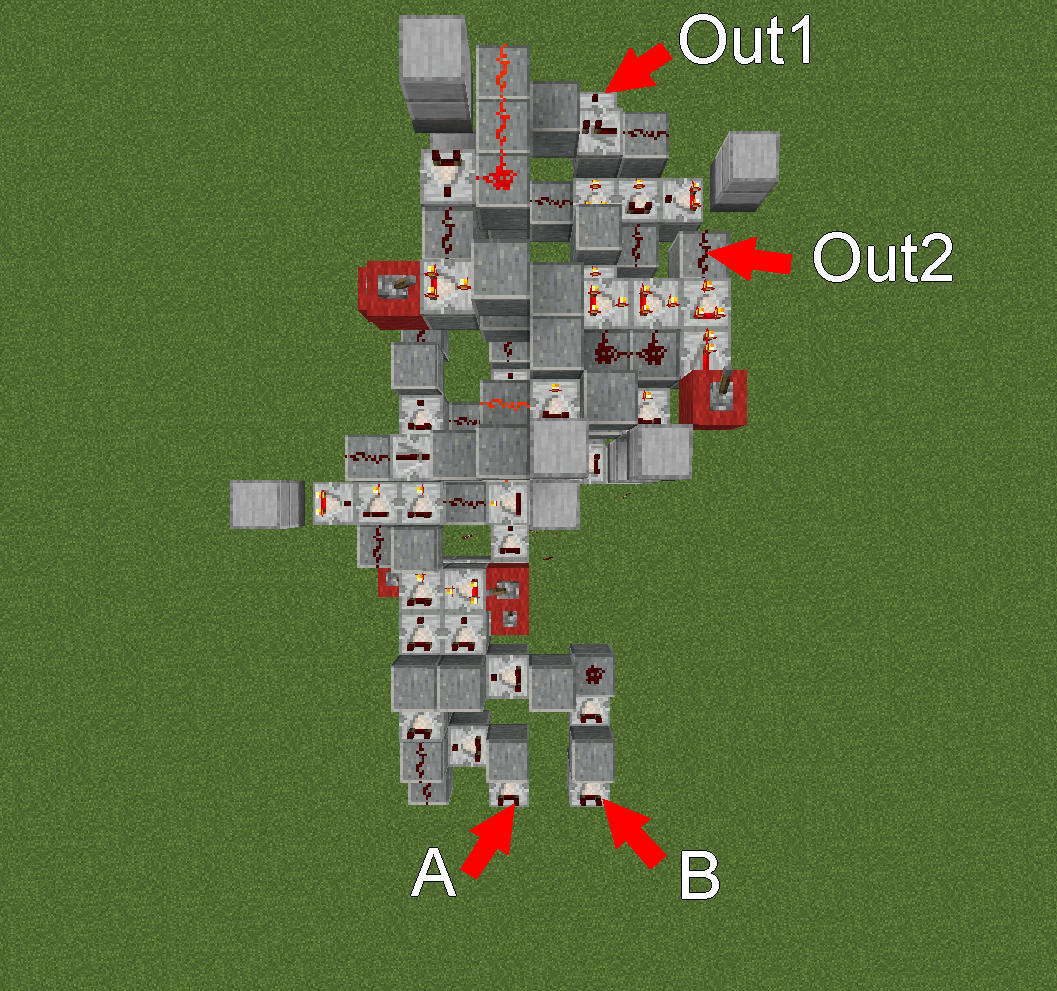How to build it , layer by layer :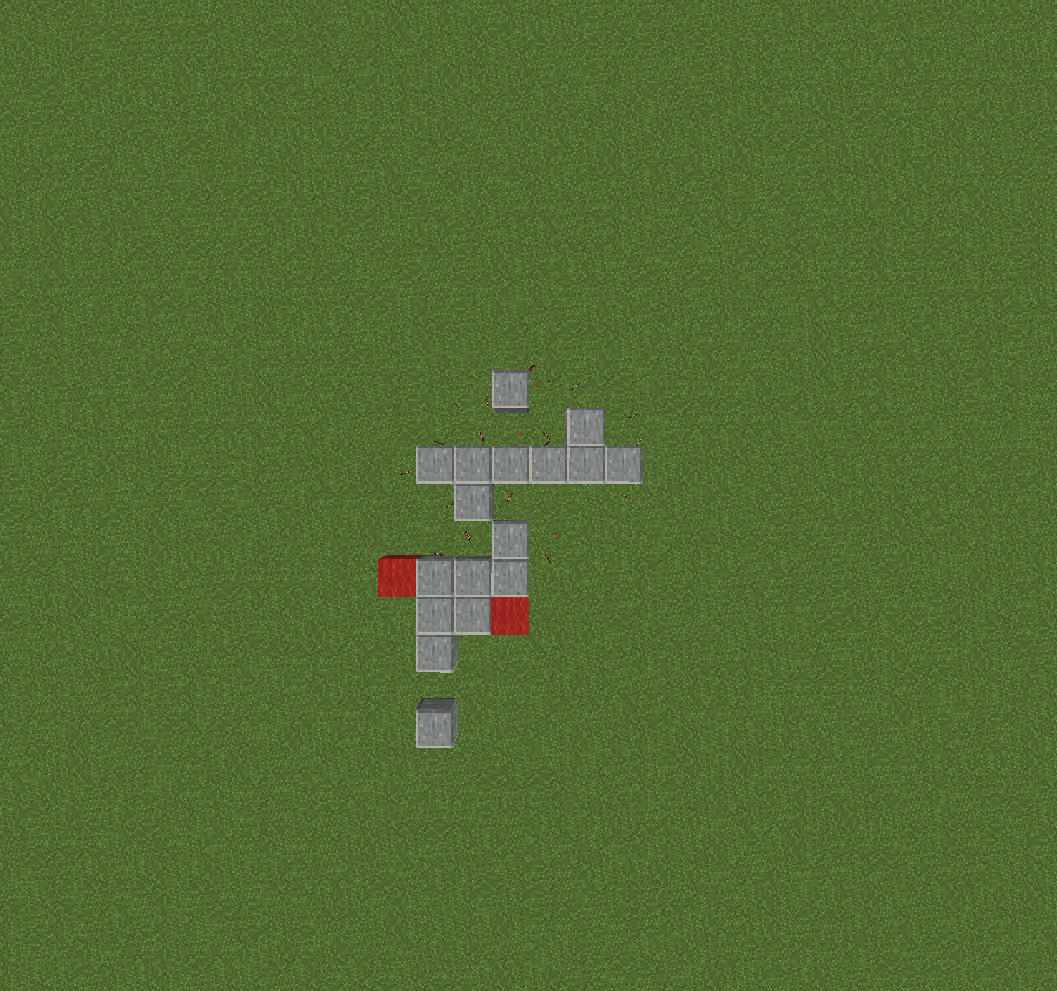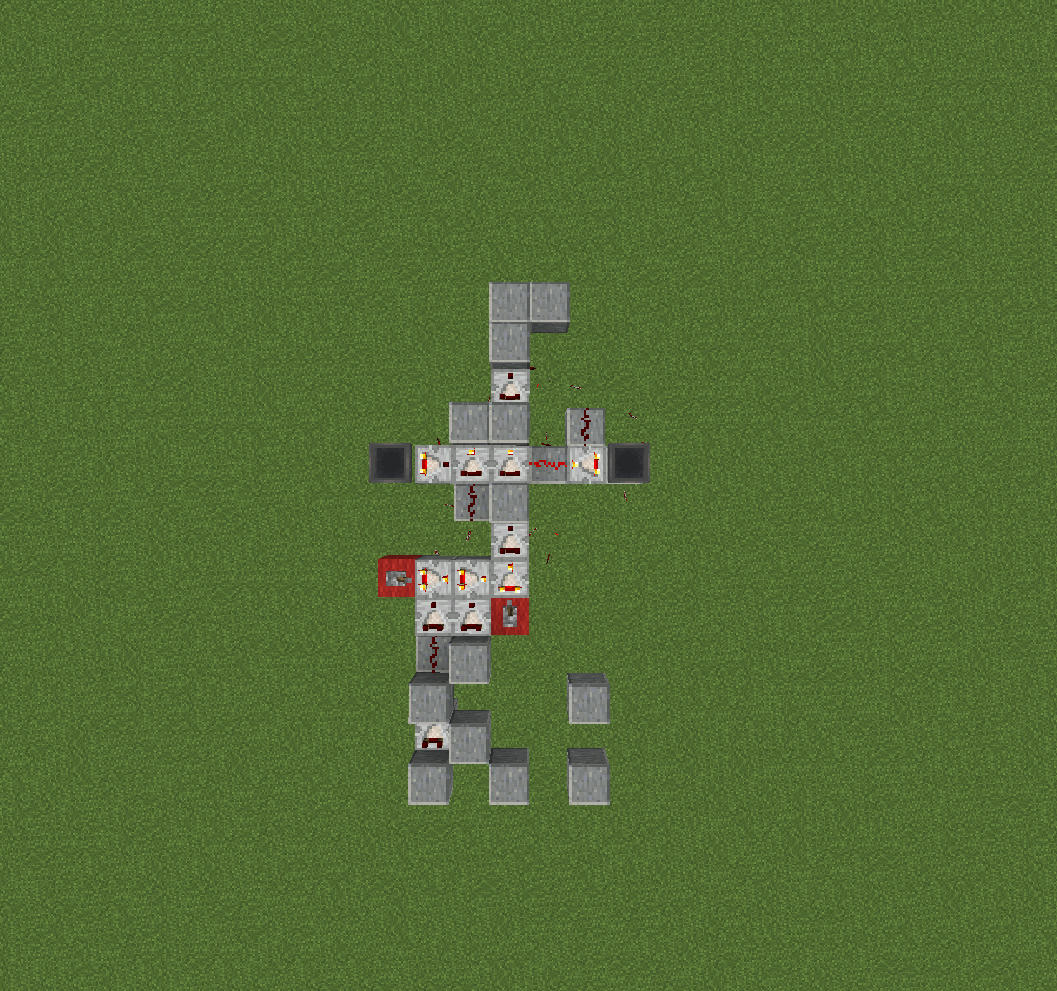hoppers : 3 * 64 items on the left , 3*64 +32 items on the right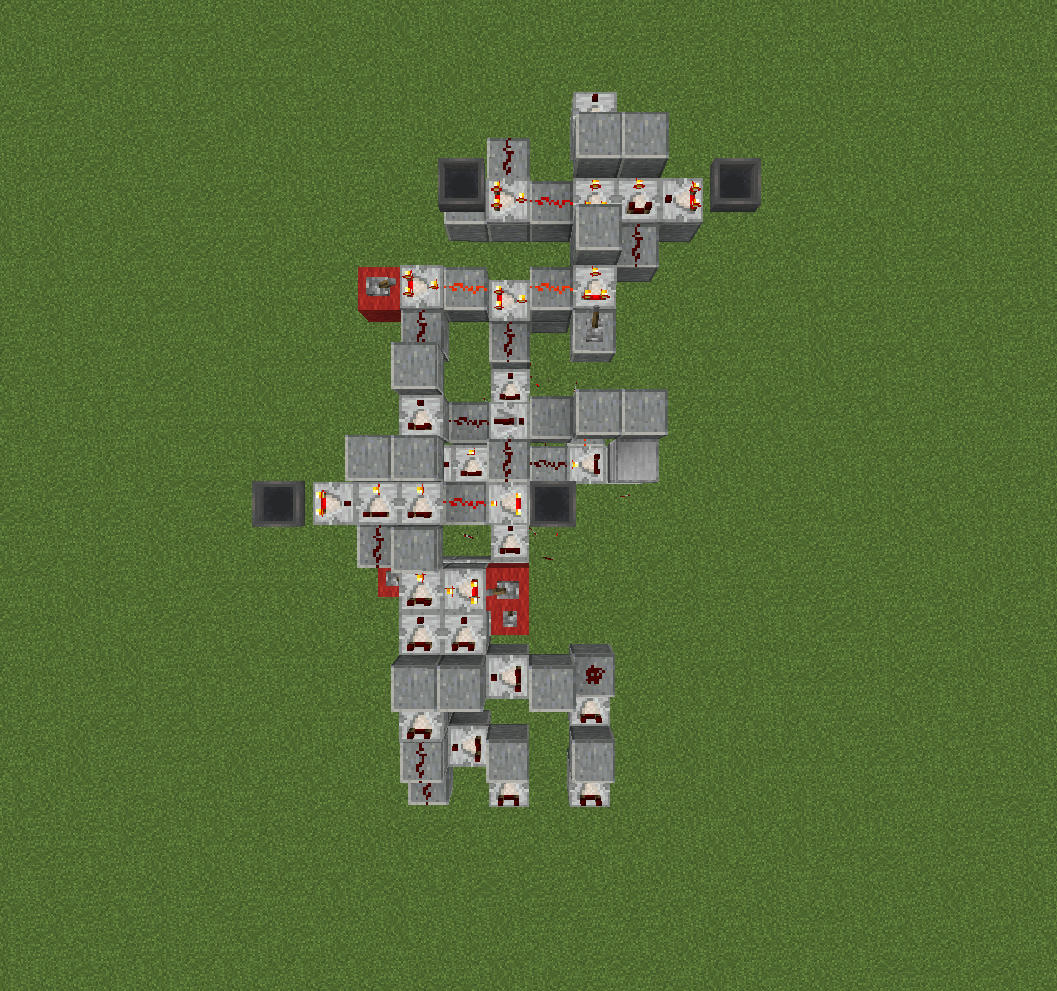hoppers at the top : 3 * 64 +32 items on the left , 3*64 items on the right
hoppers at the bottom : 3 * 64 items on the left , 3*64 +32 items on the right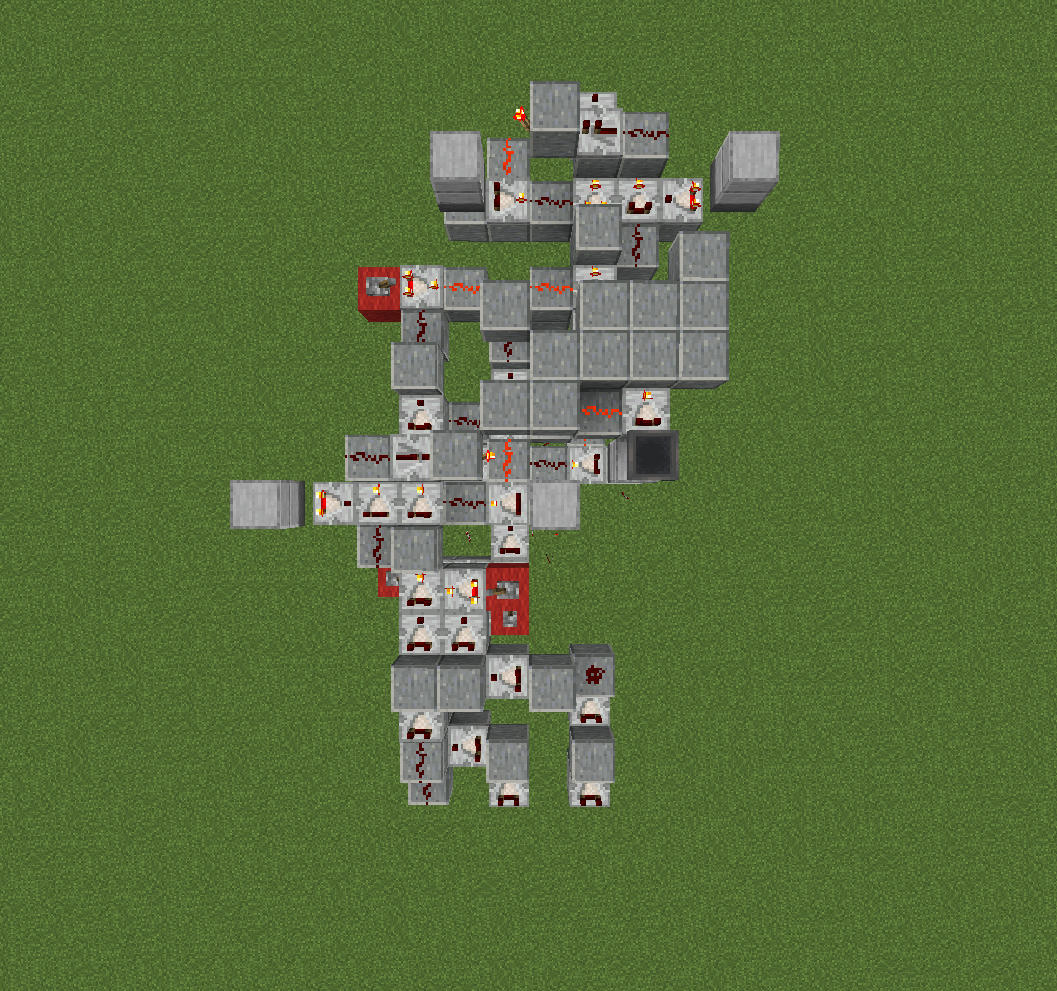hopper : 1 item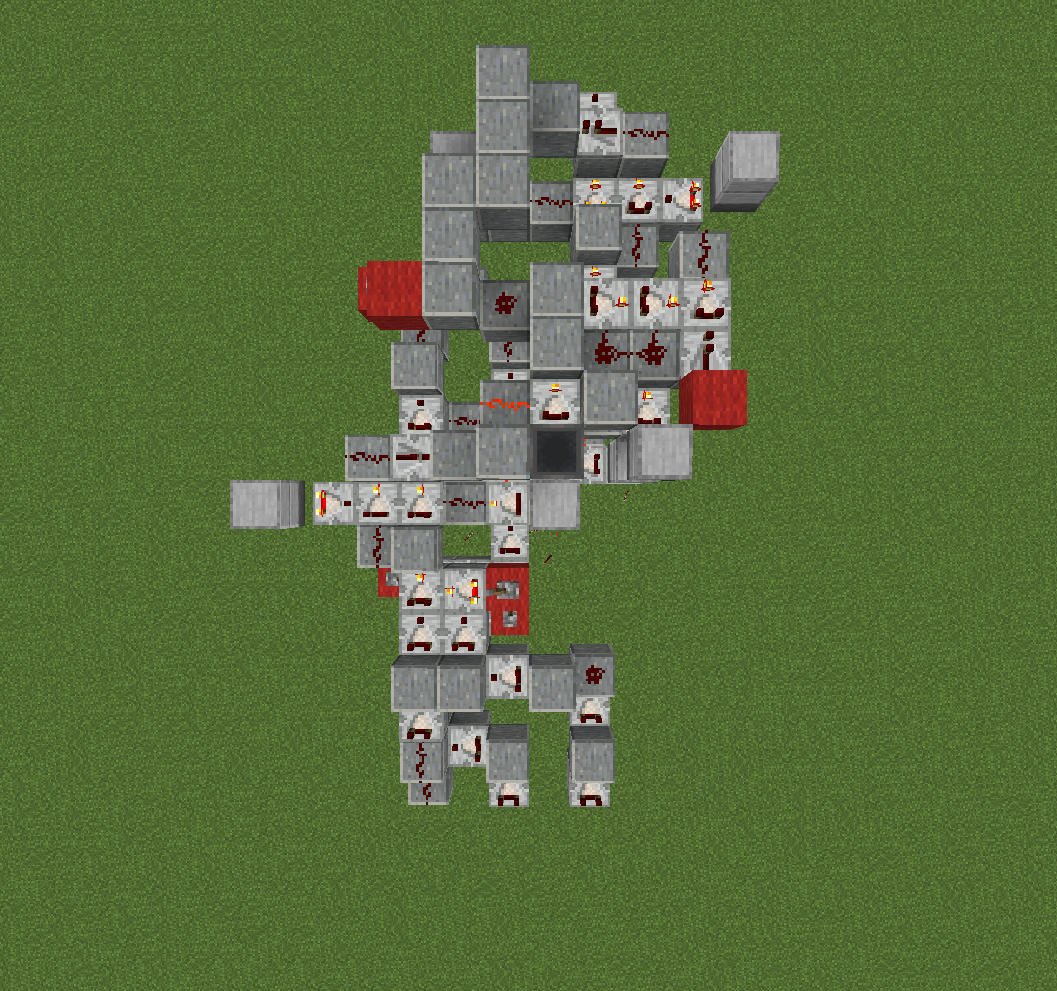hopper : 1 item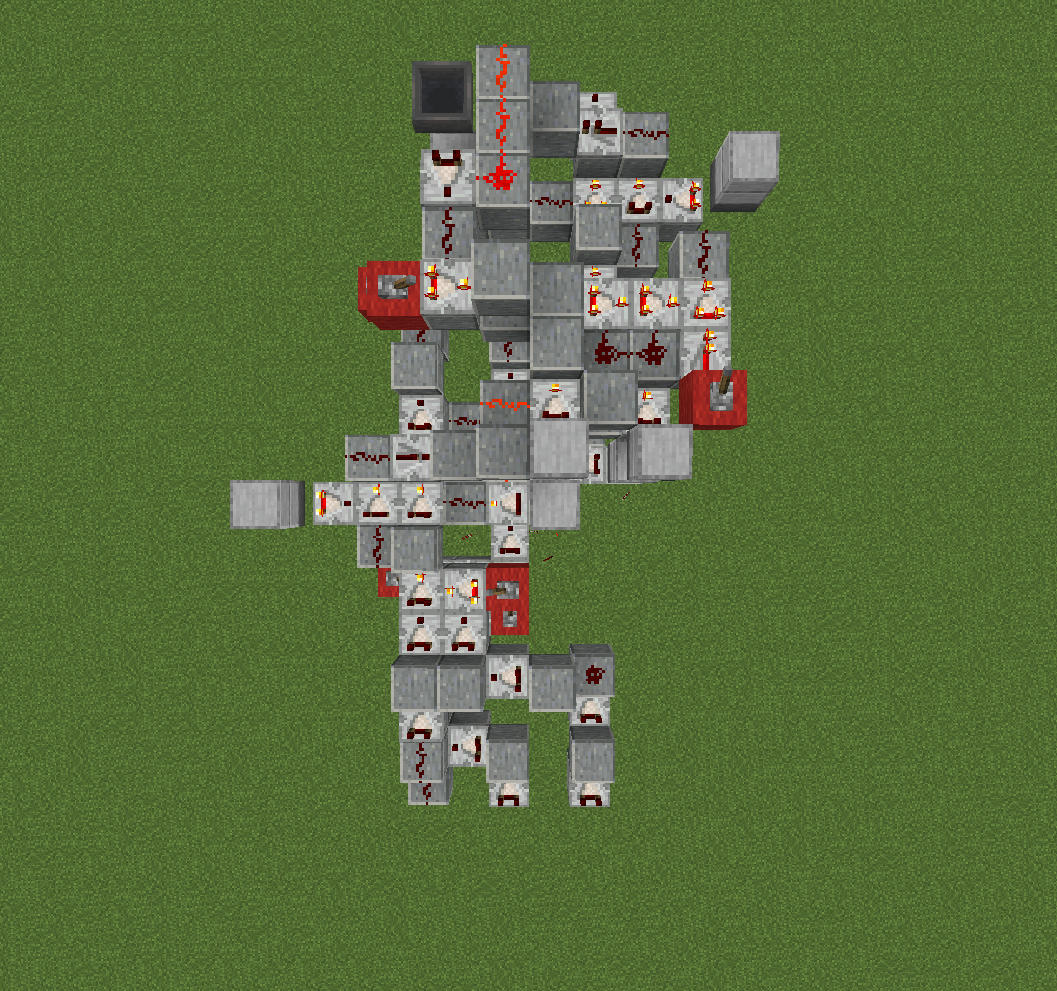hopper : 1 item

1
12/25/2021 3:29 am
Level 35 : Artisan Modder
nathan2221ok, I built this in 1.18.1 and can't get it to work. Do you happen to have a structure file of this?